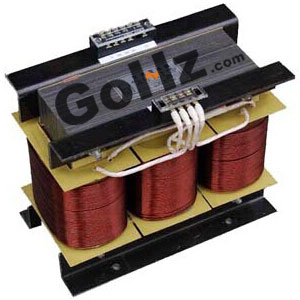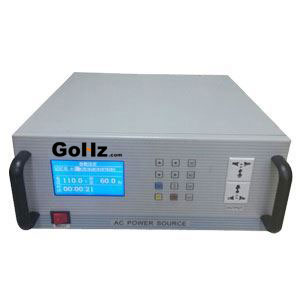Home » AC Power Source » Something about 50Hz 60Hz transformer

# Something about 50Hz 60Hz transformer

I hear comments on higher losses at the higher frequency. Derate this, derate that. All this derating talk. Comment after comment. I took some Tempel Steel M6 watts loss curves and turned them into their exponential expressions using log10 juggling to give an expression k*B^n1*f^n2. The equation is 6.1361E-6*(f)^1.6273*B^1.7784. f is in Hz, B is in kGauss. I used the 4kG curve and the 1 kG curve to derive the equation and then tried the equation out at 13.5kG and several frequencies, and it was accurate. From 50 Hz to 60 Hz, if you just plug the 50 Hz and 13.5 kGauss into the equation, and then try the equation with 60 Hz and 50/60 *14 or 11.25 kGauss, W/lb will be .365 and .355, respectively. ===>less watts loss on the core at 60Hz. What else happens?Plz Calculate (7 + 2) =
(Your comment will show after approved.)

You may also like:

50Hz 60Hz Frequency Converter Setting
Using GoHz frequency converter to
• Convert 220v 50Hz to 110v 60Hz,
• Convert 120v 60Hz to 230v 50Hz,
• Convert 110v 60Hz to 240v 50Hz,
...
• Convert 480v 60Hz to 380v 50Hz,
• Convert 400v 50Hz to 460v 60Hz,
• Convert 240v 60Hz to 380v 50Hz,
...
460v 60Hz motor on 400v 50Hz power ...Often the European motors at 1hp size are universal for 50Hz or 60Hz power supply, as long as you have 400V x 50Hz and 460V x ...
Convert 220v, 230v, 240v 50Hz to 110v, ...When you buy an 110v (120v) 60Hz appliance from USA, and run it on 220v (230v, 240v) 50Hz country (i.e. UK, Australia, ...
60Hz motor running on 50Hz power ...Electric motors, both single and three phase, are designed for running on a specified power frequency. But sometimes we may use a ...